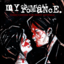MathematicsAsleepAndUnafraid21:

URGENT PLZ HELP NOW Part C: Find the distance from B to E and from P to E. Show your work. (4 points)AsleepAndUnafraid21:

here is the imageAsleepAndUnafraid21:

@aqual @uhhhkhakis plz helpUhhhKhakis:

I'm sorry fam I'm bad at math.AsleepAndUnafraid21:

oh okayAsleepAndUnafraid21:

@fortish @hunter3506 plz helpFortish:

@uhhhkhakis wrote:
I'm sorry fam I'm bad at math.
Same xdAqual:

I'm looking nowAsleepAndUnafraid21:

tyAqual:

Fist you're going to give the perimeter of cgrpAqual:

So use $p = 2(a+b)$Aqual:

p + 2(side + base)Aqual:

p = 2(400 + 600)AsleepAndUnafraid21:

so 2,000Aqual:

YesAqual:

And I realized I'm dropping that won't, my badAqual:

Perimeter isn't necessaryAsleepAndUnafraid21:

oh okAqual:

@aqual wrote:
And I realized I'm dropping that won't, my bad
Doing*Aqual:

Wrong*AsleepAndUnafraid21:

I belive i'm supposed to be using proportions but idk where to startAqual:

Is angle cgr congruent to bpeAsleepAndUnafraid21:

i think soAsleepAndUnafraid21:

aren't they alternative interior angles?Aqual:

so line segment cb is in the same position as line segment, or side, bpAsleepAndUnafraid21:

yesAqual:

so to find the change, you take side cb and divide the length by that of side bpAsleepAndUnafraid21:

I'm confused. Is the person above me right?Aqual:

yesAqual:

hold onAsleepAndUnafraid21:

but i dont understand how pbc(a line segmen) is similar to an angle @surjithayerAqual:

pbc is a triangleAqual:

or an angleAqual:

depending on how you write itAsleepAndUnafraid21:

How? pbc is on the same linesurjithayer:

if we assume GRPC is a parallelogram. Then triangles GCB and PEB are similar. $\frac{ GB }{ BE }=\frac{ GC}{ PE }=\frac{ CB }{ PB}$ $\frac{ 450 }{ BE }=\frac{ 400 }{ PE }=\frac{ 350 }{ 250 }$ $350BE=450 \times 250$ $BE=\frac{ 450\times250 }{ 350 }=\frac{ 2250 }{ 7 }=321\frac{ 3 }{7 }$ $350 PE=400\times 250=100,000$ $PE=\frac{ 100,000 }{ 350}=\frac{ 2000 }{ 7}=285\frac{ 5 }{ 7 }$AsleepAndUnafraid21:

pbe is a triangle/angleAqual:

@asleepandunafraid21 wrote:
pbe is a triangle/angle
yesAqual:

so how i do it is i take the number the triangl;e is dilated by, here its 350/250, and i divide the other side lengths to find those of the triangle im trying to findsurjithayer:

it was a mis print ,now i have corrected. thanksAsleepAndUnafraid21:

that makes more senseAqual:

so 350/250=1.4 so 450/1.4 should give you line beAqual:

400/1.4 should give you line peAsleepAndUnafraid21:

oh i think i get itAsleepAndUnafraid21:

thank you @aqual @surjithayerAqual:

@surjithayer wrote:
if we assume GRPC is a parallelogram. Then triangles GCB and PEB are similar. $\frac{ GB }{ BE }=\frac{ GC}{ PE }=\frac{ CB }{ PB}$ $\frac{ 450 }{ BE }=\frac{ 400 }{ PE }=\frac{ 350 }{ 250 }$ $350BE=450 \times 250$ $BE=\frac{ 450\times250 }{ 350 }=\frac{ 2250 }{ 7 }=321\frac{ 3 }{7 }$ $350 PE=400\times 250=100,000$ $PE=\frac{ 100,000 }{ 350}=\frac{ 2000 }{ 7}=285\frac{ 5 }{ 7 }$
these are correctAqual:

@asleepandunafraid21 wrote:
thank you @aqual @surjithayer
npsurjithayer:

yw

Latest QuestionsSailor: So. I'm gonna send out another plea for help, and I'm desperate at this point. I need to know what I did wrong here.
2 hours ago 7 Replies 3 Medalslink1234: what yall think? aye aye aye juice is in wooden box i thought u were gonna shot em first its a different story when ur poppin the percs my demons beatin me
1 hour ago 9 Replies 2 MedalsSailor: Could I get help on this? I know that absolute value should be "6th grade knowledge" but this is Algebra 2 we're talking about.
7 hours ago 1 Reply 1 MedalEvrythngizcosha: Part A. Describe in words how you can find the rate of change of a bushel of corn in the current here and find the value.
3 hours ago 1 Reply 0 Medalstim3906: Revise the passage by changing all of the gerunds to infinitives and all of the infinitives to gerunds.
6 hours ago 1 Reply 0 Medals100k: I need help
16 hours ago 2 Replies 1 MedalChrisGarcia16: Drabbles p.2 #1 we were random, we were chaotic, we were humorous. we were just children.
9 minutes ago 13 Replies 3 Medalsjay1233: What is it that lives if it is fed, and dies if you give it a drink?
1 day ago 3 Replies 1 Medaljay1233: What gets wetter the more it dries?
17 hours ago 1 Reply 1 Medalsolodive: Made a crappy album cover because I can't afford photoshop and I had to draw all of this with a mouse.
1 day ago 4 Replies 1 Medal